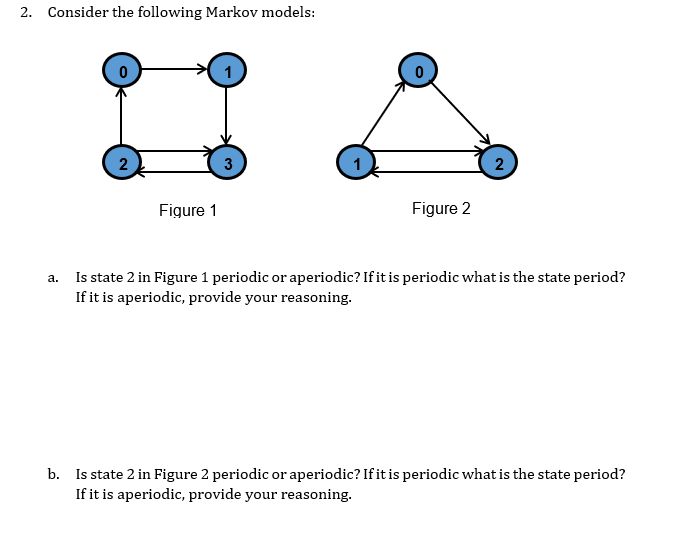Home / Expert Answers / Computer Science / 2-consider-the-following-markov-models-a-is-state-2-in-figure-1-periodic-or-aperiodic-if-it-is-pa820

# (Solved): 2. Consider the following Markov models: a. Is state 2 in Figure 1 periodic or aperiodic? If it is ...2. Consider the following Markov models: a. Is state 2 in Figure 1 periodic or aperiodic? If it is periodic what is the state period? If it is aperiodic, provide your reasoning. b. Is state 2 in Figure 2 periodic or aperiodic? If it is periodic what is the state period? If it is aperiodic, provide your reasoning.

We have an Answer from Expert